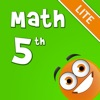# iTooch 5th Grade Math - By eduPad Inc. - Lumos Educational App Store4.51
Price -Free
\$0

#### DESCRIPTION:

With more than 4,500 exercises, iTooch Math Grade 5 is a new and fun way of practicing and learning Math for 5th Graders. It is, by far, the largest collection of educational activities based on the US National Common Core Standards on the App Store. Used by more than 4,000,000 users, iTooch apps provide comprehensive learning solutions which help parents, teachers and students to identify and address learning needs in a fun and motivating way. == UNIQUE SET OF FEATURES == Ã¢â¬Â£ A clear, simple and colorful interface to engage children to learn with fun Ã¢â¬Â£ Speech synthesis

#### OVERVIEW:

ITooch 5th Grade Math - By eduPad Inc. is a free educational mobile app By eduPad Inc..It helps students in grades 5 practice the following standards 5.OA.A.2,5.NF.A.1,5.MD.A.1.

1. 5.OA.A.2 : Write simple expressions that record calculations with numbers, and interpret numerical expressions without evaluating them. For example, express the calculation "add 8 and 7, then multiply by 2" as 2 × (8 + 7). Recognize that 3 × (18932 + 921) is three times as large as 18932 + 921, without having to calculate the indicated sum or product..

2. 5.NF.A.1 : Add and subtract fractions with unlike denominators (including mixed numbers) by replacing given fractions with equivalent fractions in such a way as to produce an equivalent sum or difference of fractions with like denominators. For example, 2/3 + 5/4 = 8/12 + 15/12 = 23/12. (In general, a/b + c/d = (ad + bc)/bd.) .

3. 5.MD.A.1 : Convert among different-sized standard measurement units within a given measurement system (e.g., convert 5 cm to 0.05 m), and use these conversions in solving multi-step real world problems..

5

#### STANDARDS:

5.OA.A.2
5.NF.A.1
5.MD.A.1
5.G.A.1
5.G.A.2

Software Version: 6.5.2

Category: Education

### RELATED APPSEdSearch WebSearch